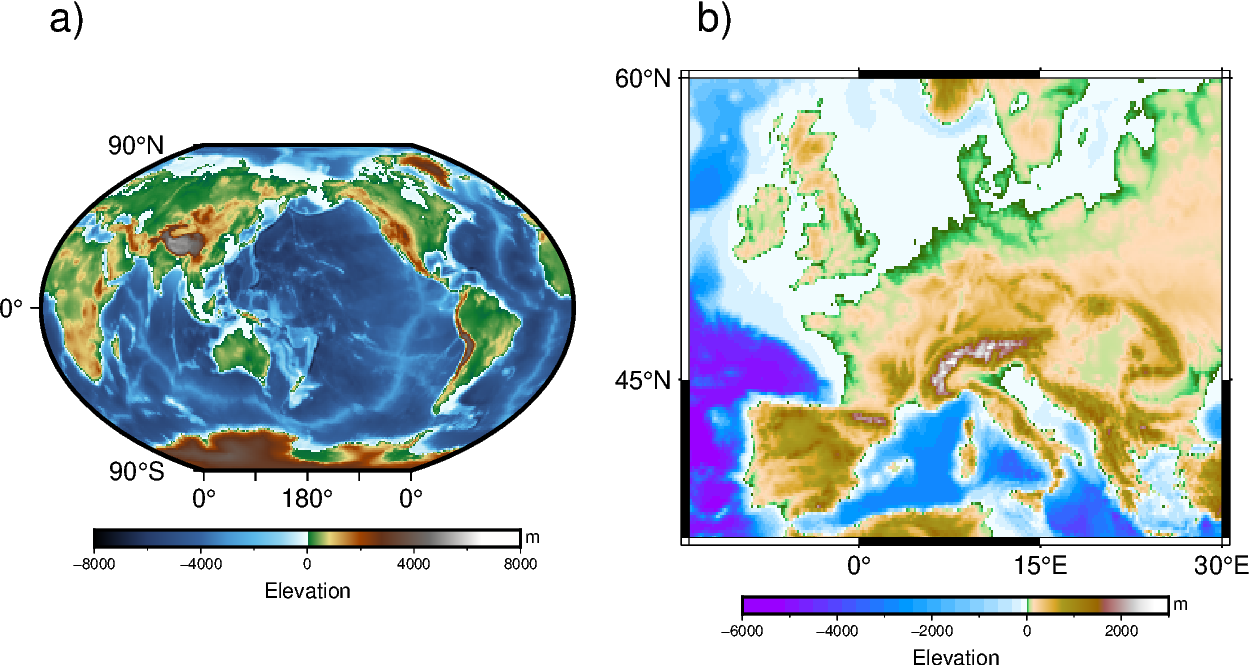# Multiple colormaps

This gallery example shows how to create multiple colormaps for different subplots. To better understand how GMT modern mode maintains several levels of colormaps, please refer to https://docs.generic-mapping-tools.org/latest/cookbook/features.html#gmt-modern-mode-hierarchical-levels for details.```import pygmt

fig = pygmt.Figure()

# Load Earth relief data for the entire globe and a subset region
subset_region = [-14, 30, 35, 60]

# Define a 1-row, 2-column subplot layout. The overall figure dimensions is set
# to be 15 cm wide and 8 cm high. Each subplot is automatically labelled.
# The space between the subplots is set to be 0.5 cm.
with fig.subplot(
nrows=1, ncols=2, figsize=("15c", "8c"), autolabel=True, margins="0.5c"
):
# Activate the first panel so that the colormap created by the makecpt
# function is a panel-level CPT
with fig.set_panel(panel=0):
pygmt.makecpt(cmap="geo", series=[-8000, 8000])
# "R?" means Winkel Tripel projection with map width automatically
# determined from the subplot width.
fig.grdimage(grid=grid_globe, projection="R?", region="g", frame="a")
fig.colorbar(frame=["a4000f2000", "x+lElevation", "y+lm"])
# Activate the second panel so that the colormap created by the makecpt
# function is a panel-level CPT
with fig.set_panel(panel=1):
pygmt.makecpt(cmap="globe", series=[-6000, 3000])
# "M?" means Mercator projection with map width also automatically
# determined from the subplot width.
fig.grdimage(grid=grid_subset, projection="M?", region=subset_region, frame="a")
fig.colorbar(frame=["a2000f1000", "x+lElevation", "y+lm"])

fig.show()
```

Total running time of the script: ( 0 minutes 1.677 seconds)

Gallery generated by Sphinx-Gallery